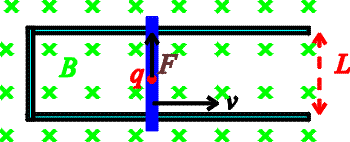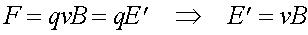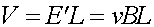Motion-induced EMF The most peculiar aspect of magnetic forces is that they act only on moving bodies. Since an object's velocity is dependent on the observer this seems paradoxical. It was mentioned previously that the paradox is the result of some very complex consequences of the theory of special relativity - that if a stationary observer sees only a magnetic field and infers a force on a moving charge due to it's velocity, an observer moving with the charge measures the charges velocity to be zero, therefore he must think there exists an electric field to account for the force. So, first consider the setup below. It consists of a current loop where the right-hand segment of the loop consists of a metallic bar which slides along the conducting rails.The force felt by a charge moving with the blue bar would be F = qvB. However, if I am an observer on the bar, there must be the same upward force even though I don't believe the charge has any velocity. I must see the same force which is proportional to the charge, and therefore I must believe there is an electric field E'.This moving observer therefore sees an electric field the stationary observer does not see. This upwards force acting over the distance L gives and effective voltage.This voltage is called an EMF which stands for electromotive force even though it is a voltage not a force! Often this voltage is given the symbol, a script "E". Since there is a voltage around the loop a current will flow due to moving the bar.